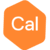# I am making a best effort to utilize the IP extension...but having some difficulties.Posts: 6Admittedly I am dealing with time-domain IP data which I normally avoid. What I don't understand is what precisely is in the array channel? I am importing gdd data with 20 gates on the decay. The channels are noted to be IP and IP_average. So what is this IP? I want chargeability which is an integral or for digital data is M=((V(t0)x(^t0)+V(t1)x(^t1)+V(t19)x(^t19))/Vp; with Vp in volts, V(t) in mvolts and ^tn is the nth window with in seconds. So what is in the array channel listed as 'IP'? The help menu says it is V(t)/Vp and that the IPaverage is (V(t0)+V(t1)+...V(t19))/nVp where n would be 20 in this case. What I want is chargeability and I would like to use something close to the 'Newmont standard', which is from 450ms to 1100 ms or thereabouts. What gives?

•Posts: 6